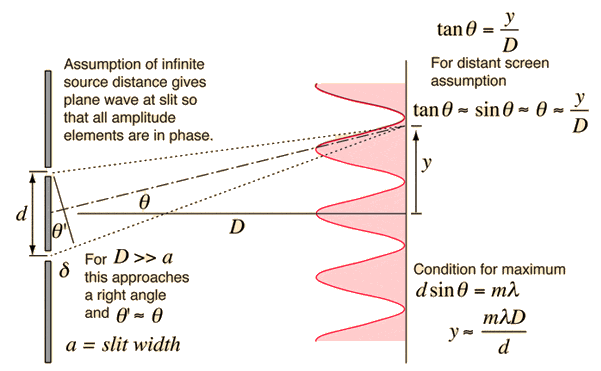# When you heat a sheet, does it expand or compress?

If a sheet containing two very thin slits is heated (without damaging it), what happens to the angular location of the first-order interference minimum?

a) It moves toward the centerline.
b) It moves away from the centerline.
c) It doesn't change.

Condition for m-order interference minimum

dsinθ=(m+1/2)λ

Given this condition, the first order condition its just m=1, thus

dsinθ=3λ/2

so, the angular location is,

θ = arcsin(3λ/2d)

Heating the sheet should cause the sheet expand, this imply the value d (distance between slits) it should be reduced. Therefore, the value of θ it should be increased, which means the answer should be letter b) It moves away from the centerline.

However, the student i tried to help told me this is incorrect, the correct answer (he/she's not sure why) is letter a) It moves toward the centerline. But this doesn't make any sense for me, can anyone give me a clue about what am i missing? Thanks in advance.

Nidum
Gold Member
If the sheet with the slits is uniformly heated and not constrained in any way then the whole sheet will expand uniformly . This means that the slits get further apart and wider . The amount of movement though will be very small .

•James Ray and Miguel Velasquez
Your
If a sheet containing two very thin slits is heated (without damaging it), what happens to the angular location of the first-order interference minimum?

a) It moves toward the centerline.
b) It moves away from the centerline.
c) It doesn't change.

Condition for m-order interference minimum

dsinθ=(m+1/2)λ

Given this condition, the first order condition its just m=1, thus

dsinθ=3λ/2

so, the angular location is,

θ = arcsin(3λ/2d)

Heating the sheet should cause the sheet expand, this imply the value d (distance between slits) it should be reduced. Therefore, the value of θ it should be increased, which means the answer should be letter b) It moves away from the centerline.

However, the student i tried to help told me this is incorrect, the correct answer (he/she's not sure why) is letter a) It moves toward the centerline. But this doesn't make any sense for me, can anyone give me a clue about what am i missing? Thanks in advance.

What I don't fully understand is what the angular location of the first-order interference minimum is.

i think theta is the angle shown in the figuresource of figure: http://hyperphysics.phy-astr.gsu.edu/hbase/phyopt/imgpho/doubsli.gif

i think theta is the angle shown in the figuresource of figure: http://hyperphysics.phy-astr.gsu.edu/hbase/phyopt/imgpho/doubsli.gif
Theta is normal to the centreline, if you define the centreline to be the axis parallel to the two sheets. Does that help answer your problem?

If the sheet with the slits is uniformly heated and not constrained in any way then the whole sheet will expand uniformly . This means that the slits get further apart and wider . The amount of movement though will be very small .

Oh now i can see where i am mistaken, the value of d is infact increasing when the sheet expand and not being reduced as i thought. This explains why the correct answer is letter a) it moves toward the centerline. When the value of d is increased, the argument of arcsin will be reduced. For some reason i've got confused about what happens to value "d" when the sheet is heated. This solves the problem. Thanks for the answer Nidum.

Theta is normal to the centreline, if you define the centreline to be the axis parallel to the two sheets. Does that help answer your problem?

Thanks for reply my question James, problem is solved. Taking again a look at the figure you can notice the value of d actually increases when the sheet is being heated which would reduce the value of theta.